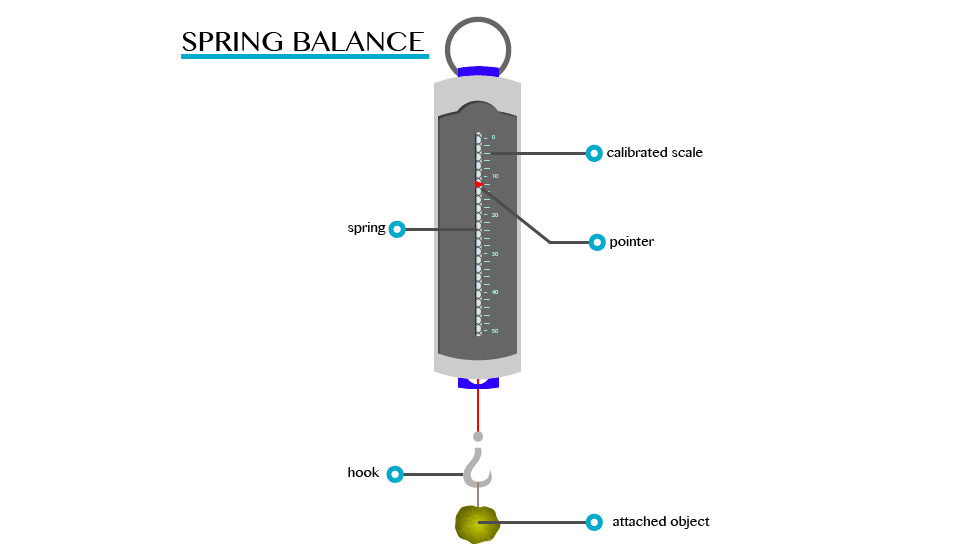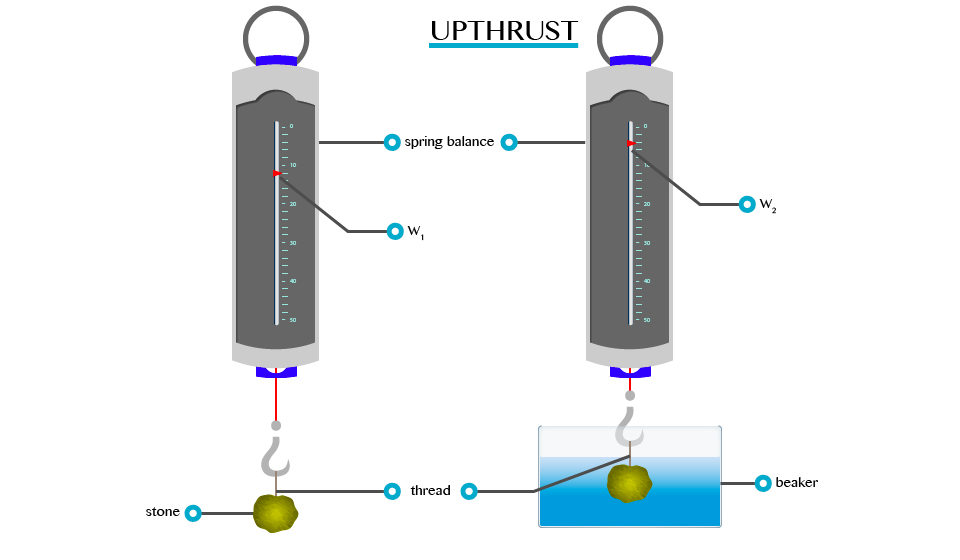# FORM ONE PHYSICS STUDY NOTES TOPIC 4-6.

1
411TOPIC 4: FORCE

Force
A
force is an interaction that causes a change. In mechanics, a force is
an interaction that causes a change in velocity (an interaction that
causes acceleration).
The Concept of Force
Explain the concept of force
For better understanding of this concept, let’s ask ourselves the following question:
1. What causes/makes a body at rest to move?
2. What causes the same body in motion to stop?
The
answers to these questions, is of course that a force is required to do
so. Here, an applied force to a body can cause the body at rest to move
or if already moving a body application of force can do the following
when applied to a body.
1. Force can cause a change in the way the object moves
2. Change its size or shape
3. Change the direction in which an object is moving
A
force is defined as a push or pulls experienced by an object. The force
is usually described in terms of its size (magnitude) and direction.
The S.I Unit of Force
State the S.I unit of force
The SI unit of force is a Newton (N).
A Newton (1N) can be defined as the amount of force required to give a mass of one kilogram (1kg) an acceleration of 1m/s2
Measuring Instrument (Device)
The instrument that is used to measure the amount of force that is exerted on an object is called spring balance.
A
spring balance can be used to measure small forces. It consists of a
coiled spring fixed to the other end with a hook at the other end. The
body upon which the force acts is attached to the hook. The distance
through which the spring is stretched is directly proportional to the
force applied by the balance.
A pointer is attached to the spring and the force is indicated on a calibrated scale.TOPIC 5: ARCHIMEDES’ PRINCIPLE AND LAW OF FLOTATION

Archimedes’
principle indicates that the upward buoyant force that is exerted on a
body immersed in a fluid, whether fully or partially submerged, is equal
to the weight of the fluid that the body displaces.
The Concept of Upthrust
Explain the concept of upthrust
If
a heavy object is lifted while immersed in water, it may be raised more
easily than when outside the water. This is due to the reason that when
anything is placed in liquid, it receives an upward force called
upthrust. A body appears to have lost some weight when immersed in water
due to the upthrust exerted on the body by the water.
• By definition upthrust is referred as an upward force exerted by the body when it’s partially or totally immersed in water.
• Consider the experiment below to verity the concept of upthrust.
From this experiment, it will be observed that W1>W2. This is because:When a body is partially or totally immersed in any liquid, the liquid exerts an upward force.
A
weight recorded on the spring balance of a body that is totally or
partially immersed in any liquid is called apparent weight. E.g. W2 and the force, which temporally reduces the weight of the body, are called upthrust (u).
Verification of the Archimedes’ Principle
Verify the archimedes principle
This
is the principle which shows the relationship between the upthrust
acting on a body and the weight of fluid it displaces when partially or
completely immersed in the fluid. It was first discovered by a Greek
scientist called Archimedes (287 to 121 BC).
• The
principle states that, ”when a body is partially or totally immersed in
a fluid it experiences an upthrust which is equal to the weight of the
fluid displaced.”
• This principle can be verified by the following experiment.
Results:Weight of a body in air = W1Verification of the Archimedes’ Principle
Weight of a body in water = W2 (apparent weight)
Uptrust = Loss of weight in water
U = W1 –W2
Example 1
Weight of a body in air = 10.0N
Weight of a body when immersed in water = 9.2N find the upthrust.
Solution:
Data given
Weight of a body in air (W1)= 10.0N
Weight of a body when in water (W2) = 9.2N
Formula
Upthrust = Loss of weight in water = W1-W2
= 10.0N – 9.2N
= 0.8N
The upthrust is 0.8
Example 2
The
weight of a body when totally immersed in liquid is 4.2N. if the weight
of the liquid displaced is 2.5N, find the weight of the body in the
air.
Solution;
Data;
Apparent weight (W2) = 4.2N
Weight of liquid displaced (u) = 2.5N
Weight of body in air=?
Formular;
U = W1 –W2
W1= 4.2 +2.5 = 6.7N
Weight of body in air is 6.7N
The Archimedes’ Principle in Determining Relative Density
Apply the archimedes principle to determine relative density
Relative
density (R.D) of a substance can be defined as a ratio of the mass of a
certain volume of the substance to the mass of an equal volume of
water.
Relative density = Mass or weight of given volume of a substance overMass or weight of an equal volume of water.
R.D = weight of a substance in air over Weight of displaced water.
R.D = weight of a substance in air
From
Archimedes’ principle the weight of an equal volume of water is equal
to the weight of water displaced by the object, which is equal to the
upthrust loss in weight. By weighing an object in air and then in water,
the relative density can be determined.
Weight of a body in air = W1
Weight of a body in water = W2
Apparent loss in weight = W1-W2
Relative density = W1/W1 – W2
Example 3
A piece of glass weigh 1.2N in air and 0.7N when completely immersed water. Calculate its:
1. Relative density
2. Density of a glass
Given that density of water = 1000kg/cm3
And acceleration due to gravity = 10N/kg
Solution
Weight of the glass in air (W1) = 1.2N
Weight of the glass in water (W2) = 0.7N
R.D = W1/W1 – W2
1.2N/(1.2 – 0.7)
1.2/0.5
R.D = 2.4
R.D = Density of glass/Density of water
Density of a glass = R.D x Density of water
= 2.4 x 1000kg/cm
= 2400kg/m3
NB: Relative density has no SI unit

#### 1 COMMENT

1. Wonderful!! Asanti sana!!